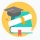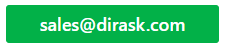Home
IT Knowledge
IT Career
Inspiration
Languages
EN

# TypeScript - constructor overloads / multiple constructors / many constructors

7 points
Created by:AnnLen
9180

## 1. Problem

Needed two or more contructors.

``````class MyClassName {

public constructor(a : number);
public constructor(a : number, b : number);
}``````

## 2. Solution

In TypeScript constructor overloading looks different way than in C++, Java or C#. Main idea to overload constructor is to create common constructor that checks what kind of parameters were passed to create object and later do some logic for proper case. Useful is to add constructors definitions to help other programmers to know how to use class in proper way.

### 2.1. Optional argument example

In this section  `?` operator were used to create common contructor.

``````class MyClassName {

//public constructor(a: number);
//public constructor(a: number, b: number);

public constructor(a: number, b?: number) {

if (b === undefined) {
console.log('Used constructor 1');
} else {
console.log('Used constructor 2');
}
}
}``````

### 2.2. Common constructor example

In this section we used array to describe common constructor.

``````class MyClassName {

public constructor(a : number);
public constructor(a : number, b : number);

public constructor(...args: any[]) {

if (args.length === 1) {
console.log('Used constructor 1');
return;
}
if (args.length === 2) {
console.log('Used constructor 2');
return;
}
throw new Error('Undefined constructor.');
}
}``````

### 2.3. Argument with multiple type example

In this section we used argument with two types.

``````class MyClassName {

//public constructor(a: number, b: number);
//public constructor(a: number, b: string);

public constructor(a: number, b: number | string) {

if (typeof b === 'number') {
console.log('Used constructor 1');
} else {
console.log('Used constructor 2');
}
}
}``````

## 3. More complicated example

Constructor overloading requires to write some logic to detect which constructor has been used. `public constructor(...args : Array<any>)` contains common logic where depending of used arguments special case of object creating should be used. Using array in common contructor we are able to use totally different constructors with different types on same place.

``````class Point {
private coordinates : Array<number>;

public constructor(x: number, y: number);
public constructor(x: number, y: number, z: number);
public constructor(x: number, y: number, z: number, ...coordinates: Array<number>);
public constructor(coordinates: Array<number>);

public constructor(...args: Array<any>) { // common logic constructor

if (args.length === 0) {
throw new Error('Arguments are not defined.');
}
let arg = args;
if (Array.isArray(arg)) {
if(arg.length < 2) {
throw new Error('Minimum number of dimmensions is 2.');
}
this.coordinates = arg;
} else {
this.coordinates = args;
}
}

public getCoordinate(dimmension: number): number {
return this.coordinates[dimmension];
}

public toString(): string {
let result = '{';
if (this.coordinates.length > 0) {
result += ' ' + this.coordinates;
for (let i = 1; i < this.coordinates.length; ++i) {
result += ', ' + this.coordinates[i];
}
}
return result + ' }';
}
}

let a = new Point(1, 2);
let b = new Point(1, 2, 3);
let c = new Point(1, 2, 3, 4);
let d = new Point([1, 2]);
let e = new Point([1, 2, 3]);
let f = new Point([1, 2, 3, 4]);

console.log(a.toString());
console.log(b.toString());
console.log(c.toString());
console.log(d.toString());
console.log(e.toString());
console.log(f.toString());``````

Output:

``````{ 1, 2 }
{ 1, 2, 3 }
{ 1, 2, 3, 4 }
{ 1, 2 }
{ 1, 2, 3 }
{ 1, 2, 3, 4 }``````

Note: big number of overloading can lead to big amount of mistakes so it is better to avoid this technic.

## 4. References